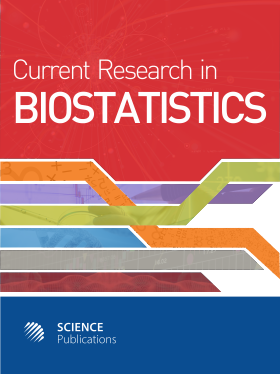Frequency: Continuous
ISSN: 2524-2210 (Print)
ISSN: 2524-2229 (Online)
Research Article Open Access

# Improvement of Bayesian Credible Interval for a Small Binomial Proportion Using Logit Transformation

Toru Ogura1 and Takemi Yanagimoto2
• 1 Mie University Hospital, Japan
• 2 Institute of Statistical Mathematics, Japan

## Abstract

A novel credible interval of the binomial proportion is proposed by improving the Highest Posterior Density (HPD) interval using the logit transformation. It is constructed in two steps: first the HPD interval for the logit transformation of the binomial proportion is driven and then the corresponding credible interval of the binomial proportion is calculated by the inverse logit transformation of that interval. Two characteristics of the proposed credible interval are: (i) the lower limit is over 0% when the zero events are obtained and (ii) the error probability is not large for any population binomial proportion. The characteristic in (i) corresponds to the claims in the Rule of Three, which means that even if zero events are obtained from n trials, the events might occur three times in other n trials. The characteristic in (ii) is important for medical research. This is because the error probabilities of all groups do not increase even if there is a high population binomial proportion among some groups. The proposed credible interval is compared with the existing confidence and credible intervals. We verified using numerical and practical examples to confirm the potential usefulness of the proposed credible interval.

Current Research in Biostatistics
Volume 8 No. 1, 2018, 1-8

DOI:

Submitted On: 23 October 2018 Published On: 20 November 2018

How to Cite: Ogura, T. & Yanagimoto, T. (2018). Improvement of Bayesian Credible Interval for a Small Binomial Proportion Using Logit Transformation. Current Research in Biostatistics, 8(1), 1-8. https://doi.org/10.3844/amjbsp.2018.1.8

• 2,690 Views